1. /
2. CBSE
3. /
4. Class 06
5. /
6. Mathematics
7. /
8. NCERT Solutions for Class...

# NCERT Solutions for Class 6 Maths Exercise 14.1

NCERT solutions for Maths Practical geometry## NCERT Solutions for Class 6 Maths Practical geometry

###### Question 1.Draw a circle of radius 3.2 cm.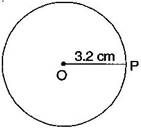(a) Open the compass for the required radius of 3.2 cm.

(b) Make a point with a sharp pencil where we want the centre of circle to be.

(c) Name it O.

(d) Place the pointer of compasses on O.

(e) Turn the compasses slowly to draw the circle.

It is required circle.

###### Question 2.With the same centre O, draw two circles of radii 4 cm and 2.5 cm.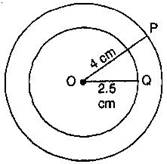(a) Marks a point ‘O’ with a sharp pencil where we want the centre of the circle.

(b) Open the compasses 4 cm.

(c) Place the pointer of the compasses on O.

(d) Turn the compasses slowly to draw the circle.

(e) Again open the compasses 2.5 cm and place the pointer of the compasses on D.

(f) Turn the compasses slowly to draw the second circle.

It is the required figure.

NCERT Solutions for Class 6 Maths Exercise 14.1

###### Question 3.Draw a circle and any two of its diameters. If you join the ends of these diameters, what is the figure obtained if the diameters are perpendicular to each other? How do you check your answer?

(i) By joining the ends of two diameters, we get a rectangle. By

measuring, we find AB = CD = 3 cm, BC = AD = 2 cm, i.e., pairs of opposite sides are equal and also {tex}\angle {/tex}A = {tex}\angle {/tex}B = {tex}\angle {/tex}C = {tex}\angle {/tex}D = {tex}{90^ \circ },{/tex} , i.e. each angle is of {tex}{90^ \circ }.{/tex}

Henc,e it is a rectangle.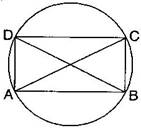(ii) If the diameters are perpendicular to each other, then by

joining the ends of two diameters, we get a square.

By measuring, we find that AB = BC = CD = DA = 2.5 cm, i.e., all four sides are equal.

Also {tex}\angle {/tex}A = {tex}\angle {/tex}B = {tex}\angle {/tex}C = {tex}\angle {/tex}D = {tex}{90^ \circ },{/tex} , i.e. each angle is of {tex}{90^ \circ }.{/tex}

Hence, it is a square.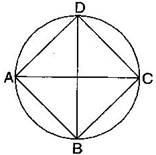NCERT Solutions for Class 6 Maths Exercise 14.1

###### Question 4.Draw any circle and mark points A, B and C such that:

(a) A is on the circle.

(b) B is in the interior of the circle.

(c) C is in the exterior of the circle.

(i) Mark a point ‘O’ with sharp pencil where we want centre of the circle.

(ii) Place the pointer of the compasses at ‘O’. Then move the compasses slowly to draw a circle.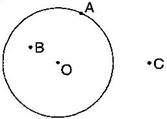(a) Point A is on the circle.

(b) Point B is in interior of the circle.

(c) Point C is in the exterior of the circle.

NCERT Solutions for Class 6 Maths Exercise 14.1

###### Question 5.Let A, B be the centres of two circles of equal radii; draw them so that each one of them passes through the centre of the other. Let them intersect at C and D. Examine whether {tex}\overline {{\text{AB}}} {/tex} and {tex}\overline {{\text{CD}}} {/tex} are at right angles.

Answer: Draw two circles of equal radii taking A and B as their centre such that one of them passes through the centre of the other. They intersect at C and D. Join AB and CD.

Yes, AB and CD intersect at right angle as {tex}\angle {/tex}COB is {tex}{90^ \circ }.{/tex}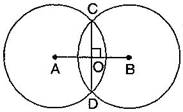## NCERT Solutions for Class 6 Maths Exercise 14.1

NCERT Solutions Class 6 Maths PDF (Download) Free from myCBSEguide app and myCBSEguide website. Ncert solution class 6 Maths includes text book solutions from Class 6 Maths Book . NCERT Solutions for CBSE Class 6 Maths have total 14 chapters. 6 Maths NCERT Solutions in PDF for free Download on our website. Ncert Maths class 6 solutions PDF and Maths ncert class 6 PDF solutions with latest modifications and as per the latest CBSE syllabus are only available in myCBSEguide.

## CBSE app for Students

To download NCERT Solutions for Class 6 Maths, Social Science Computer Science, Home Science, Hindi English, Maths Science do check myCBSEguide app or website. myCBSEguide provides sample papers with solution, test papers for chapter-wise practice, NCERT solutions, NCERT Exemplar solutions, quick revision notes for ready reference, CBSE guess papers and CBSE important question papers. Sample Paper all are made available through the best app for CBSE students and myCBSEguide website.Test Generator

Create question paper PDF and online tests with your own name & logo in minutes.myCBSEguide

Question Bank, Mock Tests, Exam Papers, NCERT Solutions, Sample Papers, Notes

### 13 thoughts on “NCERT Solutions for Class 6 Maths Exercise 14.1”

1. Good for students to learn

2. Thank you! for the third question that, I really got many help from this question.

3. Good for students to learn

4. Good for students to learn and study

5. Thank you for the answers

6. Very Useful For Students To learn , Great ?

7. Nice solution

8. Very good

9. Thank you!

10. Very good it is easily to do looser student difficult question

11. Nice solution very happy.????

12. Very nice

13. This app is best to learn maths
THANK YOU